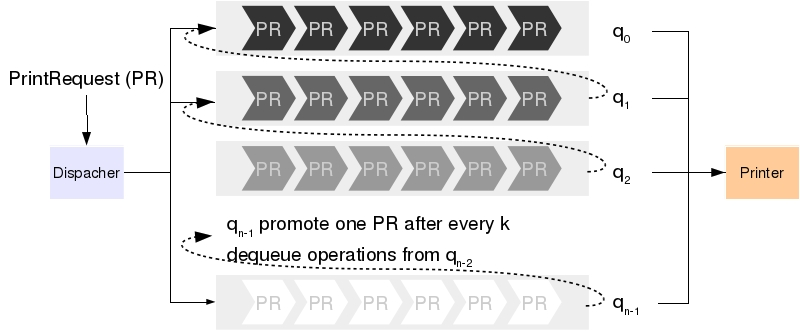# Project 7.b 打印机服务程序

## 背景• 任何时刻, 用户都可以向服务器发送打印请求
• 每个请求包含打印文件的文件名字, 页数.
• 服务器根据文件页数初始化该请求的优先级, 并将该打印请求放入对应的队列中.
• 具体的初始化优先级算法见后续描述
• 如果打印机空闲, 则从优先级最高的非空队列中选择请求进行打印
• 如果打印机忙, 则让其继续打印
• 当一个打印任务完成, 服务器将进行以下操作
• 如果所有队列为空, 则等待新的任务到来.
• 如果存在队列非空, 则从优先级最高的非空队列中选择一个请求. 同时, 如果一个队列 $$q_i$$ 已经被访问过 $$k$$ 次, 则选择优先级最高非空队列 $$q_j, j > i$$, 从 $$q_j$$ 中选择一个任务将其放入 $$q_i$$ 中, 并清空 $$q_i$$的访问次数.

1. 所有请求赋予相同优先级, 放入 $$q_0$$ 中. 等价于只有一个队列的 FIFS.
2. 使用 n 个队列, 根据文档页数分配优先级
• 页数在 1 到 10 之间, 则进入队列 $$q_0$$
• 页数在 11 到 20, 则进入队列 $$q_1$$
• ...
• 页数在 10(n-2)+1 到 10(n-1) 之间, 则进入队列 $$q_{n-2}$$
• 页数超过 10(n-1), 则进入队列 $$q_{n-1}$$
3. 使用 n 个队列, 若文件页数为 t, 则进入队列 $$q_{t\%n}$$

## 实验内容

1. 定义 PrintRequest 类表示打印请求. 需要包含: 文件的名称, 页数以及发出该请求时的时间.

2. 定义 Printer 类表示打印机. 至少包含以下方法
• boolean printerIdle(): 如果打印机空闲返回 true, 否则返回 false
• boolean printFile(PrintRequest r): 如果打印机空闲, 则开始处理打印任务 r, 并返回 true. 否则忽略改请求, 返回 false.
• PrintRequest processForOneUnit(), 如果打印机空闲, 或者当前正在打印的文档还剩余超过1页则返回 null. 如果当前文档已完成, 则返回当前的打印任务对象.
3. 定义 PrintQueue 类表示每一个队列, 实现 Queue 接口 (提示: 队列可能需要记录当前已经被访问了多少次).

4. 定义 PrintDispatcher 类实现 Queue 接口. 包含 n 个 PrintQueue 对象, 完成上述"动态优先级"策略. 包含 toString() 方法可以显示逐个队列的状态.

5. 定义 PrintSimulation 类, 模拟打印场景.
• 从命令行接收4个参数, 分别
• p: 当前这1秒钟有打印请求到来的概率
• n: 使用多少个队列
• k: 当某个队列被访问了 k 次后, 将提升优先级
• a: 选择哪种初始化优先级的策略 (A, B, C)
• 模拟每一秒系统的行为. 可能出现的情况为
• 如果打印机当前正忙, 则打印当前任务的一页.
• 同时, 以概率 p 产生一个打印请求. 该请求的文档页数为 1 到 100 间的随机数, 发出请求的时间为当前 clock 变量的值. 该打印请求被放入 PrintDispatcher 中 (通过调用enqueue() 方法).
• 如果打印机空闲, 且有请求正在等待, 则通过 PrintDispatcher 选择一个任务交给 Printer (通过调用 dequeue() 方法). 同时, 通过该任务的发出时间, 计算该任务的总共的等待时间.
• 当以上过程进行了 1000 秒后 (clock = 0, 1, ..., 999), 不再产生新的请求. 但过程将继续下去, 直到所有没有完成任务被完成.
• 输出所有任务中, 最长的等待时间和平均等待时间.
• 请绘制直方图, 横坐标表示页数 (10, 20, ..., 10(n-1), >10(n-1)), 纵坐标表示页数落在对应横坐标范围内的所有请求的平均等待时间.
6. 根据程序运行结果分析不同参数对系统的影响.